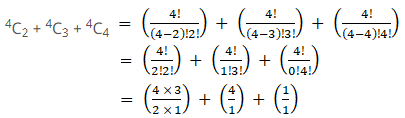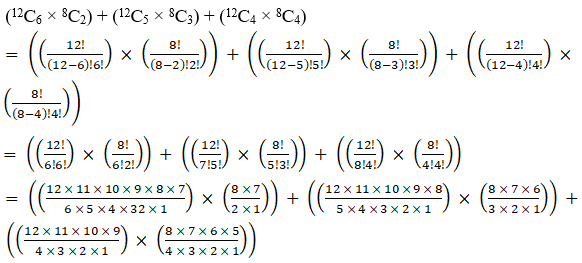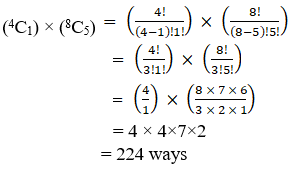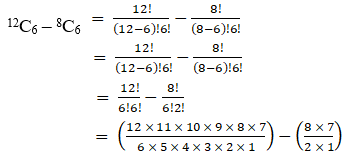• +91 9971497814
• info@interviewmaterial.com

# RD Chapter 17- Combinations Ex-17.2 Interview Questions Answers

### Related Subjects

Question 1 : From a group of 15 cricket players, a team of 11 players is to be chosen. In how many ways can this be done?

Given:

Number of players = 15

Number of players tobe selected = 11

By using the formula,

nCr = n!/r!(n – r)!

15C11 = 15! / 11! (15 – 11)!

= 15! / (11! 4!)

= [15×14×13×12×11!] /(11! 4!)

= [15×14×13×12] /(4×3×2×1)

= 15×7×13

= 1365

The total numberof ways of choosing 11 players out of 15 is 1365 ways.

Question 2 : How many different boat parties of 8, consisting of 5 boys and 3 girls, can be made from 25 boys and 10 girls?

Given:

Total boys are = 25

Total girls are = 10

Boat party of 8 to bemade from 25 boys and 10 girls, by selecting 5 boys and 3 girls.

So,

By using the formula,

nCr = n!/r!(n – r)!

25C5 × 10C3 =25!/5!(25 – 5)! × 10!/3!(10-3)!

= 25! / (5! 20!) ×10!/(3! 7!)

=[25×24×23×22×21×20!]/(5! 20!) × [10×9×8×7!]/(7! 3!)

= [25×24×23×22×21]/5!× [10×9×8]/(3!)

=[25×24×23×22×21]/(5×4×3×2×1) × [10×9×8]/(3×2×1)

= 5×2×23×11×21 × 5×3×8

= 53130 × 120

= 6375600

The total numberof different boat parties is 6375600 ways.

Question 3 : In how many ways can a student choose 5 courses out of 9 courses if 2 courses are compulsory for every student?

Given:

Total number ofcourses is 9

So out of 9 courses 2courses are compulsory. Student can choose from 7(i.e., 5+2) courses only.

That too out of 5courses student has to choose, 2 courses are compulsory.

So they have to choose3 courses out of 7 courses.

This can be donein 7C3 ways.

By using the formula,

nCr = n!/r!(n – r)!

7C3 = 7! / 3! (7 – 3)!

= 7! / (3! 4!)

= [7×6×5×4!] / (3! 4!)

= [7×6×5] / (3×2×1)

= 7×5

= 35

The total numberof ways of choosing 5 subjects out of 9 subjects in which 2 are compulsory is35 ways.

Question 4 : In how many ways can a football team of 11 players be selected from 16 players? How many of these will (i) Include 2 particular players? (ii) Exclude 2 particular players?

Given:

Total number ofplayers = 16

Number of players tobe selected = 11

So, the combinationis 16C11

By using the formula,

nCr = n!/r!(n – r)!

16C11 = 16! / 11! (16 – 11)!

= 16! / (11! 5!)

= [16×15×14×13×12×11!]/ (11! 5!)

= [16×15×14×13×12] /(5×4×3×2×1)

= 4×7×13×12

= 4368

(i) Include 2particular players?

It is told that twoplayers are always included.

Now, we have to select9 players out of the remaining 14 players as 2 players are already selected.

Number of ways = 14C9

14C9 = 14! / 9! (14 – 9)!

= 14! / (9! 5!)

= [14×13×12×11×10×9!]/ (9! 5!)

= [14×13×12×11×10] /(5×4×3×2×1)

= 7×13×11×2

= 2002

(ii) Exclude 2particular players?

It is told that twoplayers are always excluded.

Now, we have to select11 players out of the remaining 14 players as 2 players are already removed.

Number of ways = 14C9

14C11 = 14! / 11! (14 – 11)!

= 14! / (11! 3!)

= [14×13×12×11!] /(11! 3!)

= [14×13×12] / (3×2×1)

= 14×13×2

= 364

The required no.of ways are 4368, 2002, 364.

Question 5 :
There are 10 professors and 20 students out of whom a committee of 2 professors and 3 students is to be formed. Find the number of ways in which this can be done. Further, find in how many of these committees:
(i) a particular professor is included.
(ii) a particular student is included.
(iii) a particular student is excluded.

Given:

Total number ofprofessor = 10

Total number ofstudents = 20

Number of ways =(choosing 2 professors out of 10 professors) × (choosing 3 students out of 20students)

= (10C2)× (20C3)

By using the formula,

nCr = n!/r!(n – r)!

10C2 × 20C3 =10!/2!(10 – 2)! × 20!/3!(20-3)!

= 10!/(2! 8!) ×20!/(3! 17!)

= [10×9×8!]/(2! 8!) ×[20×19×18×17!]/(17! 3!)

= [10×9]/2! ×[20×19×18]/(3!)

= [10×9]/(2×1) ×[20×19×18]/(3×2×1)

= 5×9 × 10×19×6

= 45 × 1140

= 51300 ways

(i) a particular professoris included.

Number of ways =(choosing 1 professor out of 9 professors) × (choosing 3 students out of 20students)

9C1 × 20C3

By using the formula,

nCr = n!/r!(n – r)!

9C1 × 20C=9!/1!(9 – 1)! × 20!/3!(20-3)!

= 9!/(1! 8!) × 20!/(3!17!)

= [9×8!]/(8!) ×[20×19×18×17!]/(17! 3!)

= 9 × [20×19×18]/(3!)

= 9×[20×19×18]/(3×2×1)

= 9 × 10×19×6

= 10260 ways

(ii) a particular studentis included.

Number of ways =(choosing 2 professors out of 10 professors) × (choosing 2 students out of 19students)

10C2 × 19C2

By using the formula,

nCr = n!/r!(n – r)!

10C2 × 19C2 =10!/2!(10 – 2)! × 19!/2!(19-2)!

= 10!/(2! 8!) ×19!/(2! 17!)

= [10×9×8!]/(2! 8!) ×[19×18×17!]/(17! 2!)

= [10×9]/2! ×[19×18]/(2!)

= [10×9]/(2×1) ×[19×18]/(2×1)

= 5×9 × 19×9

= 45 × 171

= 7695 ways

(iii) a particular studentis excluded.

Number of ways =(choosing 2 professors out of 10 professors) × (choosing 3 students out of 19students)

10C2 × 19C3

By using the formula,

nCr = n!/r!(n – r)!

10C2 × 19C3 =10!/2!(10 – 2)! × 19!/3!(19-3)!

= 10!/(2! 8!) ×19!/(3! 16!)

= [10×9×8!]/(2! 8!) ×[19×18×17×16!]/(16! 3!)

= [10×9]/2! ×[19×18×17]/(3!)

= [10×9]/(2×1) ×[19×18×17]/(3×2×1)

= 5×9 × 19×3×17

= 45 × 969

= 43605 ways

The required no.of ways are 51300, 10260, 7695, 43605.

Question 6 : How many different products can be obtained by multiplying two or more of the numbers 3, 5, 7, 11 (without repetition)?

Given that we need tofind the no. of ways of obtaining a product by multiplying two or more from thenumbers 3, 5, 7, 11.

Number of ways = (no.of ways of multiplying two numbers) + (no. of ways of multiplying threenumbers) + (no. of multiplying four numbers)

4C2 + 4C3 + 4C4

By using the formula,

nCr = n!/r!(n – r)!= 12/2 + 4 + 1

= 6 + 4 + 1

= 11

The total numberof ways of product is 11 ways.

Question 7 : From a class of 12 boys and 10 girls, 10 students are to be chosen for the competition, at least including 4 boys and 4 girls. The 2 girls who won the prizes last year should be included. In how many ways can the selection be made?

Given:

Total number of boys =12

Total number of girls= 10

Total number of girlsfor the competition = 10 + 2 = 12

Number of ways = (no.of ways of selecting 6 boys and 2 girls from remaining 12 boys and 8 girls) + (no.of ways of selecting 5 boys and 3 girls from remaining 12 boys and 8 girls) +(no. of ways of selecting 4 boys and 4 girls from remaining 12 boys and 8girls)

= (12C6 × 8C2)+ (12C5 × 8C3) + (12C4 × 8C4)

By using the formula,

nCr = n!/r!(n – r)!= (924 × 28) + (792 ×56) + (495 × 70)

= 25872 + 44352 +34650

= 104874

The total numberof ways of product is 104874 ways.

Question 8 :
How many different selections of 4 books can be made from 10 different books, if
(i) there is no restriction
(ii) two particular books are always selected
(iii) two particular books are never selected

Given:

Total number of books= 10

Total books to beselected = 4

(i) there is norestriction

Number of ways =choosing 4 books out of 10 books

10C4

By using the formula,

nCr = n!/r!(n – r)!

10C4 = 10! / 4! (10 – 4)!

= 10! / (4! 6!)

= [10×9×8×7×6!] / (4!6!)

= [10×9×8×7] /(4×3×2×1)

= 10×3×7

= 210 ways

(ii) two particular booksare always selected

Number of ways =select 2 books out of the remaining 8 books as 2 books are already selected.

8C2

By using the formula,

nCr = n!/r!(n – r)!

8C2 = 8! / 2! (8 – 2)!

= 8! / (2! 6!)

= [8×7×6!] / (2! 6!)

= [8×7] / (2×1)

= 4×7

= 28 ways

(iii) two particular booksare never selected

Number of ways = select4 books out of remaining 8 books as 2 books are already removed.

8C4

By using the formula,

nCr = n!/r!(n – r)!

8C4 = 8! / 4! (8 – 4)!

= 8! / (4! 4!)

= [8×7×6×5×4!] / (4!4!)

= [8×7×6×5] /(4×3×2×1)

= 7×2×5

= 70 ways

The required no.of ways are 210, 28, 70.

Question 9 : From 4 officers and 8 jawans in how many ways can 6 be chosen (i) to include exactly one officer (ii) to include at least one officer?

Given:

Total number ofofficers = 4

Total number of jawans= 8

Total number ofselection to be made is 6

(i) to include exactly oneofficer

Number of ways = (no.of ways of choosing 1 officer from 4 officers) × (no. of ways of choosing 5jawans from 8 jawans)

= (4C1)× (8C5)

By using the formula,

nCr = n!/r!(n – r)!(ii) to include at leastone officer?

Number of ways =(total no. of ways of choosing 6 persons from all 12 persons) – (no. of ways ofchoosing 6 persons without any officer)

12C6 – 8C6

By using the formula,

nCr = n!/r!(n – r)!= (11×2×3×2×7) – (4×7)

= 924 – 28

= 896 ways

The required no.of ways are 224 and 896.

Question 10 : A sports team of 11 students is to be constituted, choosing at least 5 from class XI and at least 5 from class XII. If there are 20 students in each of these classes, in how many ways can the teams be constituted?

Given:

Total number ofstudents in XI = 20

Total number ofstudents in XII = 20

Total number ofstudents to be selected in a team = 11 (with atleast 5 from class XI and 5 fromclass XII)

Number of ways = (No.of ways of selecting 6 students from class XI and 5 students from class XII) +(No. of ways of selecting 5 students from class XI and 6 students from classXII)

= (20C6 × 20C5)+ (20C5 × 20C6)

= 2 (20C6 × 20C5)ways

Todays Deals### RD Chapter 17- Combinations Ex-17.2 Contributorskrishan

Name:
Email:

# Latest News# 9000 interview questions in different categories